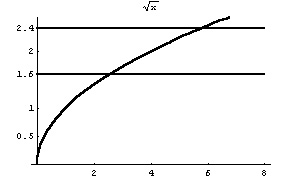LIMITS

1.  Enter the limit and sketch a graph. Show all  asymptotes.

 lim x ® 4 x-4 2x+3

2.  Find the limit. Enter the numerator first followed by the denominator:

 lim x ® a x-4 2x+3

3. Find and enter the limit. Sketch the graph paying attention to any asymptotes.

 lim x ® -3 x2-x-12 x+3

4. Prove that

 lim x ® 3 (4x-5) = 7     by finding a rule     d = d(e)

Enter the numerator of the rule followed by the denominator (use e for epsilon):

5. Find the following limit:

 lim h ® 0 (3+h)2 - 9 h
6. Enter the following limit:
 lim x ® 0+ |x| x
7. Sketch the graph of the Greatest Integer Function. Enter the limit:
 lim x ® 1+ [[x]]

8.

 Use the given graph to find  the largest   d > 0    such that     | __Ö x - 2 | < 0.4     if     0 < |x - 4| < d
 Enter d    to 2 decimal places9. Find the limit.

 lim t ® 0 Sin(5t) t

10. Find the limit and enter below:(numerator followed by denominator)
 lim x ® 0 tan(x) 3x

11. Sketch the graph. Enter the limit. Support your answer by an argument.

 lim x ® 0 x2 Sin æç è 1 x ö÷ ø

12. Find the following limit:
 lim x ® ±¥ 2x3 + 3x x3 +1